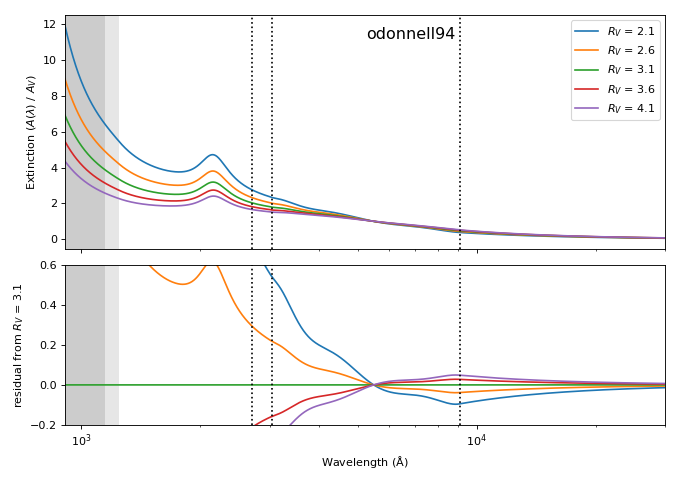# extinction¶

Fast interstellar dust extinction laws in Python

Cython-optimized implementations of empirical dust exitinction laws found in the literature.

## Installation¶

Using conda:

conda install -c conda-forge extinction


Using pip (requires a C compiler):

pip install extinction


Extinction depends on numpy and scipy.

Development version / source code: http://github.com/kbarbary/extinction

## Usage¶

Functions:

 extinction.ccm89(wave, a_v, r_v[, unit, out]) Cardelli, Clayton & Mathis (1989) extinction function. extinction.odonnell94(wave, a_v, r_v[, …]) O’Donnell (1994) extinction function. extinction.calzetti00(wave, a_v, r_v[, …]) Calzetti (2000) extinction function. extinction.fitzpatrick99(wave, a_v[, r_v, unit]) Fitzpatrick (1999) dust extinction function. extinction.fm07(wave, a_v[, unit]) Fitzpatrick & Massa (2007) extinction model for R_V = 3.1. extinction.apply(extinction, flux[, inplace]) Apply extinction to flux values. extinction.remove(extinction, flux[, inplace]) Remove extinction from flux values.

Classes:

 extinction.Fitzpatrick99 Fitzpatrick (1999) dust extinction function for arbitrary R_V.

Examples:

Get extinction in magnitudes at a set of wavelengths for various dust laws:

>>> import numpy as np
>>> import extinction

>>> wave = np.array([2000., 4000., 8000.])  # wavelength in Angstroms

# Cardelli, Clayton & Mathis (1989) with A_V = 1 and R_V = 3.1
>>> extinction.ccm89(wave, 1.0, 3.1)
array([ 2.84252644,  1.4645557 ,  0.59748901])  # extinction in magnitudes

# O'Donnell (1994)
>>> extinction.odonnell94(wave, 1.0, 3.1)
array([ 2.84252644,  1.42617802,  0.60793495])

# Fitzpatrick (1999)
>>> extinction.fitzpatrick99(wave, 1.0, 3.1)
array([ 2.76225609,  1.79674653,  1.42325373])


The Fitzpatrick & Massa (2007) function has a fixed $$R_V$$ of 3.1:

>>> extinction.fm07(wave, 1.0)
array([ 2.90478329,  1.42645161,  0.54703201])


All extinction laws accept a unit keyword to change the interpretation of the wavelength array from Angstroms to inverse microns:

>>> wave = np.array([5., 2.5, 1.25])  # wavelength in inverse microns

>>> extinction.ccm89(wave, 1.0, 3.1, unit='invum')
array([ 2.84252644,  1.4645557 ,  0.59748901])  # extinction in magnitudes


### Redden or deredden¶

To “redden” or “deredden” flux values by some amount, use the apply and remove convenience functions:

>>> from extinction import ccm89, apply

>>> flux = np.ones(3)

# "redden" flux by A_V = 1.0
>>> apply(ccm89(wave, 1.0, 3.1), flux)
array([ 0.07294397,  0.25952412,  0.5767723 ])

# "deredden" flux by A_V = 1.0
>>> remove(ccm89(wave, 1.0, 3.1), flux)
array([ 13.70915145,   3.85320647,   1.73378645])


### Comparison of functions¶### R_V dependence of odonnell94¶### R_V dependence of Fitzpatrick99¶### A note on parameterization¶

Most extinction laws here have two parameters: $$A_V$$ and $$R_V$$. $$A_V$$ is a simply a linear scaling parameter; that is: ccm89(wave, 2.0, 3.1) is the same as 2.0 * ccm89(wave, 1.0, 3.1). $$R_V$$ changes the shape of the extinction curve, rather than just the amplitude.

Traditionally, the meaning ascribed to these parameters was that $$A_V$$ is the extinction in the V band, and $$R_V$$ describes the ratio of total to selective extinction: $$R_V = A_V / E(B-V)$$, where $$E(B-V)$$ is the difference in extinction between the B and V bands. While this is approximately correct, the measured extinction of a source in actual B and V bandpasses will generally depend on the source spectrum and the shape of the specific bandpasses. Therefore, the $$A_V$$ and $$R_V$$ that are parameters in our extinction law will not correspond perfectly to measured B and V extinctions. So, in the context of these extinction laws, it is best to think of $$A_V$$ and $$R_V$$ as simply parameters that describe the amplitude and shape of the wavelength dependence, rather than corresponding directly to measured magnitudes.

Finally, for a given shape of the extinction curve (described by $$R_V$$), one can equally well use $$E(B-V)$$ as a linear scaling parameter in place of $$A_V$$, with the equivalence $$E(B-V) = A_V / R_V$$. Note that the above caution applies here: $$E(B-V)$$ should be considered simply a parameter describing amplitude of extinction; it will not correspond exactly to a difference in measured B and V extinctions.

## License and Credits¶

The license is MIT. Part of this code originated in the specutils package.